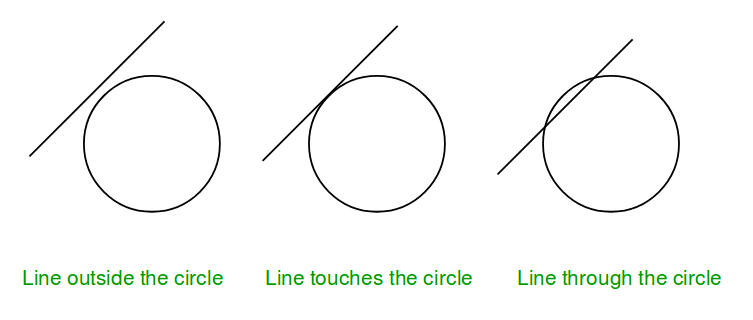Open in App
Not now

# Check if a line touches or intersects a circle

• Difficulty Level : Easy
• Last Updated : 23 Dec, 2022

Given coordinate of the center and radius > 1 of a circle and the equation of a line. The task is to check if the given line collides with the circle or not. There are three possibilities :

1. Line intersects the circle.
2. Line touches the circle.
3. Line is outside the circleNote: General equation of a line is a*x + b*y + c = 0, so only constant a, b, c are given in the input.

Examples :

Input : radius = 5, center = (0, 0),
a = 1, b = -1, c = 0.
Output : Intersect

Input :  radius = 5, center = (0, 0),
a = 5, b = 0, c = 0.
Output : Touch

Input : radius = 5, center = (0, 0),
a = 1, b = 1, c = -16.
Output : Outside

The idea is to compare the perpendicular distance between center of circle and line with the radius of the circle.
Algorithm:
1. Find the perpendicular (say p) between center of circle and given line.
2. Compare this distance p with radius r.
……a) If p > r, then line lie outside the circle.
……b) If p = r, then line touches the circle.
……c) If p < r, then line intersect the circle.
How to find the perpendicular distance?
Distance of a line from a point can be computed using below formula:Refer Wiki for details of above formula.

## C++

 // CPP program to check if a line touches or  // intersects or outside a circle. #include  using namespace std;   void checkCollision(int a, int b, int c,                    int x, int y, int radius) {     // Finding the distance of line from center.     int dist = (abs(a * x + b * y + c)) /                       sqrt(a * a + b * b);       // Checking if the distance is less than,      // greater than or equal to radius.     if (radius == dist)         cout << "Touch" << endl;     else if (radius > dist)         cout << "Intersect" << endl;     else         cout << "Outside" << endl; }   // Driven Program int main() {     int radius = 5;     int x = 0, y = 0;     int a = 3, b = 4, c = 25;     checkCollision(a, b, c, x, y, radius);     return 0; }

## Java

 // Java program to check if a line touches or  // intersects or outside a circle.   import java.io.*;   class GFG {           static void checkCollision(int a, int b, int c,                                 int x, int y, int radius)     {         // Finding the distance of line from center.         double dist = (Math.abs(a * x + b * y + c)) /                          Math.sqrt(a * a + b * b);               // Checking if the distance is less than,          // greater than or equal to radius.         if (radius == dist)             System.out.println ( "Touch" );         else if (radius > dist)             System.out.println( "Intersect") ;         else             System.out.println( "Outside") ;     }           // Driven Program     public static void main (String[] args)      {         int radius = 5;         int x = 0, y = 0;         int a = 3, b = 4, c = 25;         checkCollision(a, b, c, x, y, radius);           } }   // This article is contributed by vt_m.

## Python3

 # python program to check if a line # touches or  intersects or outside # a circle.   import math   def checkCollision(a, b, c, x, y, radius):           # Finding the distance of line      # from center.     dist = ((abs(a * x + b * y + c)) /             math.sqrt(a * a + b * b))       # Checking if the distance is less      # than, greater than or equal to radius.     if (radius == dist):         print("Touch")     elif (radius > dist):         print("Intersect")     else:         print("Outside")   # Driven Program radius = 5 x = 0 y = 0 a = 3 b = 4 c = 25 checkCollision(a, b, c, x, y, radius)   # This code is contributed by Sam007

## C#

 // C# program to check if a line touches or  // intersects or outside a circle. using System;   class GFG {           static void checkCollision(int a, int b, int c,                              int x, int y, int radius)     {         // Finding the distance of line from center.         double dist = (Math.Abs(a * x + b * y + c)) /                          Math.Sqrt(a * a + b * b);               // Checking if the distance is less than,          // greater than or equal to radius.         if (radius == dist)             Console.WriteLine ("Touch");         else if (radius > dist)             Console.WriteLine("Intersect");         else             Console.WriteLine("Outside");     }           // Driven Program     public static void Main ()      {         int radius = 5;         int x = 0, y = 0;         int a = 3, b = 4, c = 25;                   checkCollision(a, b, c, x, y, radius);           } }   // This article is contributed by vt_m.

## PHP

  $dist)  echo "Intersect";  else  echo "Outside" ; } // Driver Code $radius = 5; $x = 0; $y = 0; $a = 3; $b = 4; $c = 25; checkCollision($a, $b, $c, $x, $y, \$radius);   // This code is contributed by Sam007 ?>

## Javascript

 

Output

Touch


Time Complexity : O(log(a*a + b*b)) as it is using inbuilt sqrt function
Auxiliary Space : O(1)

This article is contributed by Anuj Chauhan. If you like GeeksforGeeks and would like to contribute, you can also write an article using write.geeksforgeeks.org or mail your article to review-team@geeksforgeeks.org. See your article appearing on the GeeksforGeeks main page and help other Geeks.